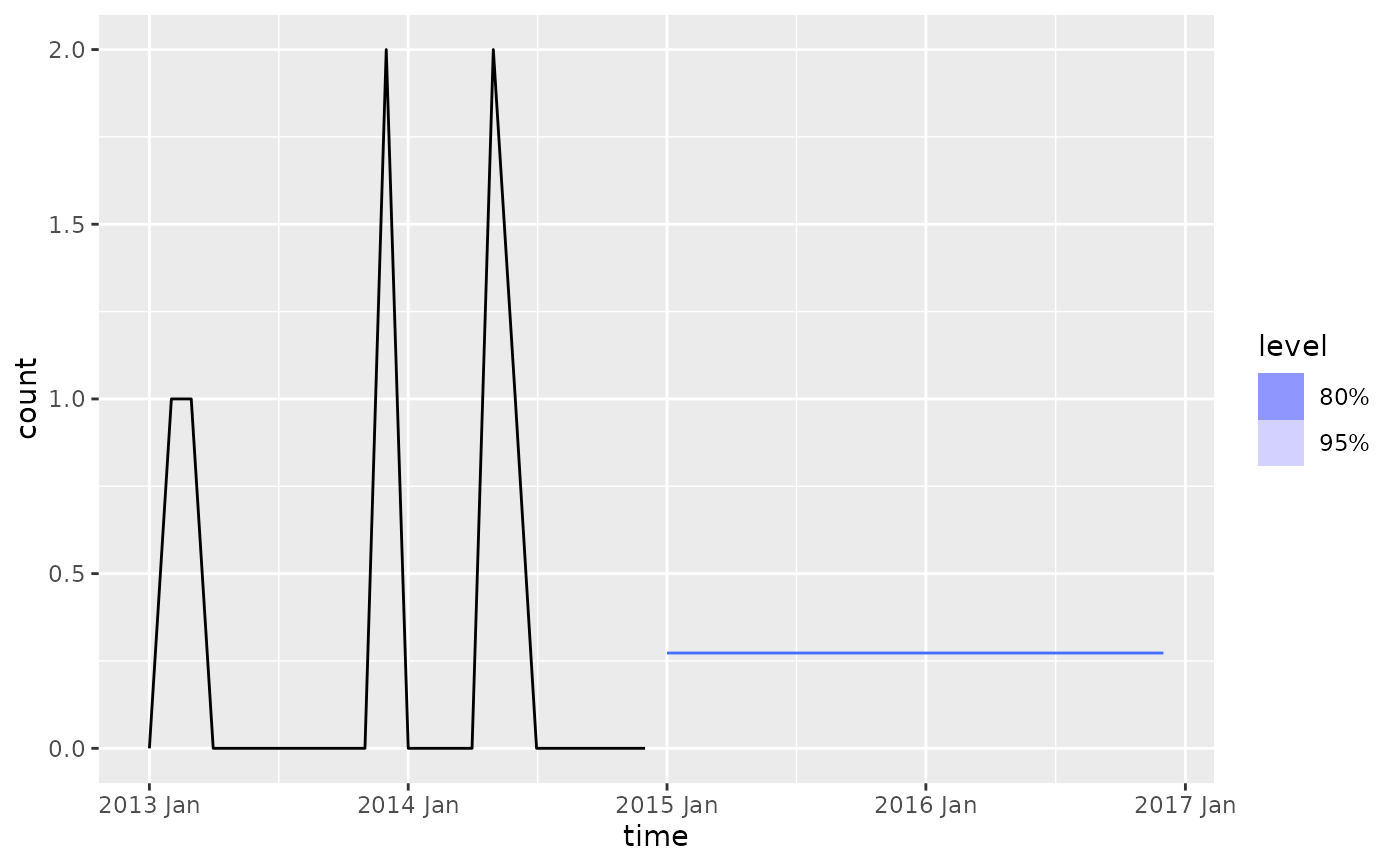Based on Croston's (1972) method for intermittent demand forecasting, also described in Shenstone and Hyndman (2005). Croston's method involves using simple exponential smoothing (SES) on the non-zero elements of the time series and a separate application of SES to the times between non-zero elements of the time series.

CROSTON(
formula,
opt_crit = c("mse", "mae"),
type = c("croston", "sba", "sbj"),
...
)

## Arguments

formula Model specification (see "Specials" section). The optimisation criterion used to optimise the parameters. Which variant of Croston's method to use. Defaults to "croston" for Croston's method, but can also be set to "sba" for the Syntetos-Boylan approximation, and "sbj" for the Shale-Boylan-Johnston method. Not used.

## Value

A model specification.

## Details

Note that forecast distributions are not computed as Croston's method has no underlying stochastic model. In a later update, we plan to support distributions via the equivalent stochastic models that underly Croston's method (Shenstone and Hyndman, 2005)

There are two variant methods available which apply multiplicative correction factors to the forecasts that result from the original Croston's method. For the Syntetos-Boylan approximation (type = "sba"), this factor is $$1 - \alpha / 2$$, and for the Shale-Boylan-Johnston method (type = "sbj"), this factor is $$1 - \alpha / (2 - \alpha)$$, where $$\alpha$$ is the smoothing parameter for the interval SES application.

## Specials

### demand

The demand special specifies parameters for the demand SES application.

demand(initial = NULL, param = NULL, param_range = c(0, 1))

 initial The initial value for the demand application of SES. param The smoothing parameter for the demand application of SES. param_range If param = NULL, the range of values over which to search for the smoothing parameter.

### interval

The interval special specifies parameters for the interval SES application.

interval(initial = NULL, param = NULL, param_range = c(0, 1))

 initial The initial value for the interval application of SES. param The smoothing parameter for the interval application of SES. param_range If param = NULL, the range of values over which to search for the smoothing parameter.

Croston, J. (1972) "Forecasting and stock control for intermittent demands", Operational Research Quarterly, 23(3), 289-303.

Shenstone, L., and Hyndman, R.J. (2005) "Stochastic models underlying Croston's method for intermittent demand forecasting". Journal of Forecasting, 24, 389-402.

Kourentzes, N. (2014) "On intermittent demand model optimisation and selection". International Journal of Production Economics, 156, 180-190. doi: 10.1016/j.ijpe.2014.06.007 .

## Examples

library(tsibble)
sim_poisson <- tsibble(
time = yearmonth("2012 Dec") + seq_len(24),
count = rpois(24, lambda = 0.3),
index = time
)

sim_poisson %>%
autoplot(count)sim_poisson %>%
model(CROSTON(count)) %>%
forecast(h = "2 years") %>%
autoplot(sim_poisson)